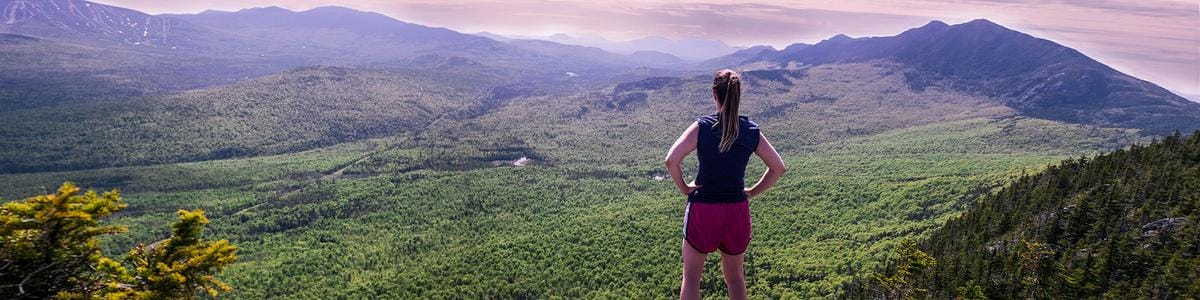Waylon Wolfe

We already think you're cool if you use Maine Trail Finder! Use your MTF account to keep track of all the trails you have visited, and Maine Trail Finder will automatically award you digital badges as you complete any of the following trail challenges.

Earning badges is all based on the honor system and your trail log reports. Did you really hike 10 miles yesterday, or did it just feel like that? It's on your conscience.

Wear your badges with pride! And don't hesitate to brag just a little bit. Use Maine Trail Finder's social media sharing links to tell your friends about your accomplishments and general awesomeness!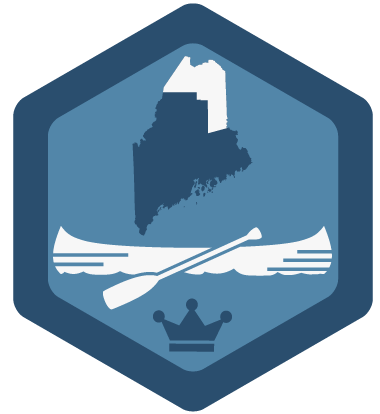Featured

Paddle two water trails in Aroostook County to be crowned with this badge.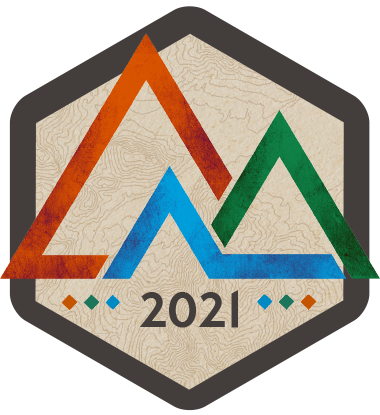Featured

### Second Nature Adventure Challenge 2021

Give back when you get outside! Give back when you get outside! Join 200 of your friends and neighbors to complete 500 trails in western Maine before September 6, 2021 and Maine West will donate \$3,000 to local after-school programs. Sign up here!### 7 in 7

Quite a stretch! You've completed seven trails in as many days!Paddle two water trails in Aroostook County to be crowned with this badge.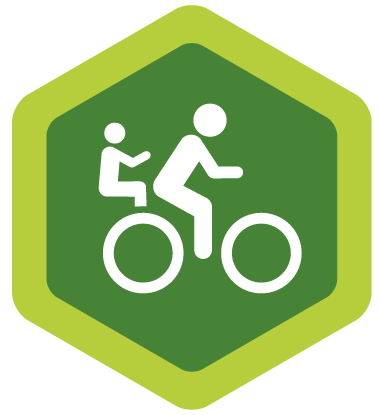### Easy Rider

Now you're rolling! You've got three paved multi-use trails under your wheels (or feet).### Fall Fanatic

Hey autumnal ace, you've logged 5 trails this fall!### Fun in the Sun

A hot weather hero. You've logged 5 trails this summer!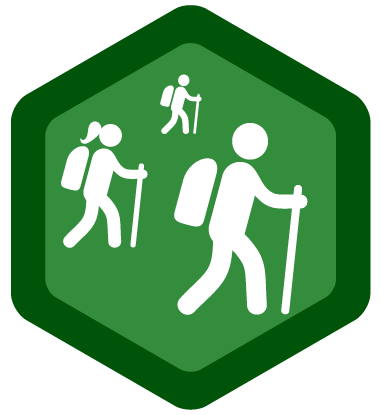### Go the (Social) Distance

Take care of your mind and body while social distancing. Use "Find Trails Near Me" to discover local trails and log 6 trail miles...while staying 6 feet apart (Trails & COVID-19)### History Buff

You're too busy to collect dust. You’ve hiked 5 trails with historical features in Maine.### Marathoner

You've gone the distance! You've logged over 26.2 trail miles!A cause to celebrate! You've logged your very first trail.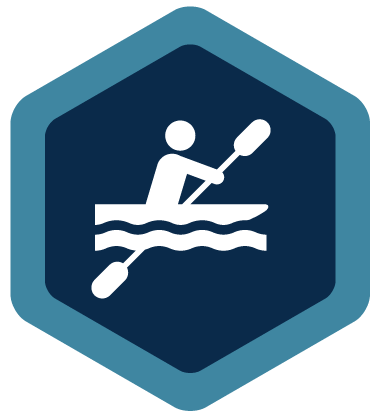### River Roamer

Paddle power! You've now completed two water trails.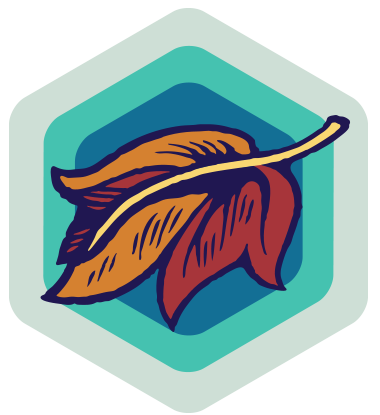### River Tripper

Brought to you by Allagash Brewing Company, celebrate Maine's mighty rivers - on water and off. Log four riverine trails and toast your adventures with a pint of River Trip!### Second Nature Adventure Challenge 2021

Give back when you get outside! Give back when you get outside! Join 200 of your friends and neighbors to complete 500 trails in western Maine before September 6, 2021 and Maine West will donate \$3,000 to local after-school programs. Sign up here!### Spring Fling

A vernal victory. You've logged 5 trails this spring!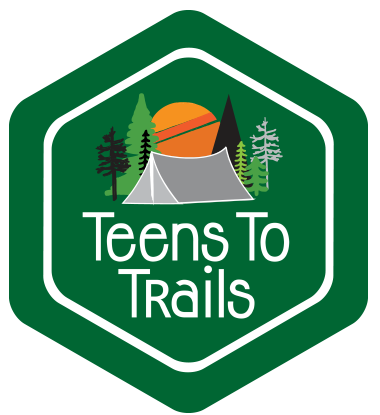### Teens to Trails Trekker

Complete any three of these favorites from Teens to Trails and discover how Life Happens Outside!### Tour de Maine

Don the yellow jersey! You've just completed 21 trails within 23 days.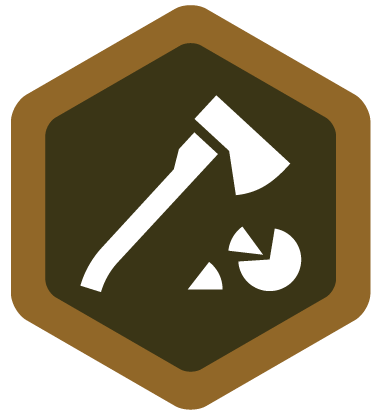### Virtuous Volunteer

We salute you! You have gotten down and dirty doing trail maintenance on at least one trail.### Winter Wanderer

Cold weather doesn't slow you down. You've logged 5 trails this winter!

+DEBUG
```Array
(
 => Array
(
[isFeatured] => 0
[description] => <p>Quite a stretch! You've completed seven trails in as many days!</p>
[image] => Array
(
[alt] => 7 in 7
[fileExtension] => png
[fileName] => _7_in_7_300-image.png
[tag] => <img src="https://cdn.branchcms.com/l4gOa2eDBE-756/images/trails/badge/_7_in_7_300-image.png" alt="7 in 7">
[type] => image
[typeName] => PNG Image
)

[imageMedium] => Array
(
[alt] => 7 in 7
[fileExtension] => png
[fileName] => _7_in_7_300-image_medium.png
[tag] => <img src="https://cdn.branchcms.com/l4gOa2eDBE-756/images/trails/badge/_7_in_7_300-image_medium.png" alt="7 in 7">
[type] => image
[typeName] => PNG Image
)

[imageLarge] => Array
(
[alt] => 7 in 7
[fileExtension] => png
[fileName] => _7_in_7_300-image_large.png
[tag] => <img src="https://cdn.branchcms.com/l4gOa2eDBE-756/images/trails/badge/_7_in_7_300-image_large.png" alt="7 in 7">
[type] => image
[typeName] => PNG Image
)

[ruleTypeKey] => completed-days
[id] => 8
[hasTrails] =>
[ruleTypeLabel] => Completed in Days
)

 => Array
(
[isFeatured] => 1
[description] => <p>Paddle two water trails in Aroostook County to be crowned with this badge.</p>
[image] => Array
(
[fileExtension] => png
[type] => image
[typeName] => PNG Image
)

[imageMedium] => Array
(
[fileExtension] => png
[type] => image
[typeName] => PNG Image
)

[imageLarge] => Array
(
[fileExtension] => png
[type] => image
[typeName] => PNG Image
)

[ruleTypeKey] => completed-pool
[id] => 25
[hasTrails] => 1
[trails] => Array
(
 => Array
(
[id] => 806
)

 => Array
(
[id] => 852
)

 => Array
(
[id] => 853
)

 => Array
(
[id] => 1241
)

 => Array
(
[id] => 1252
)

 => Array
(
[id] => 1253
)

 => Array
(
[id] => 1254
)

 => Array
(
[id] => 1255
)

 => Array
(
[id] => 1256
)

 => Array
(
[id] => 1257
)

 => Array
(
[id] => 1258
)

 => Array
(
[id] => 1259
)

 => Array
(
[id] => 1260
)

 => Array
(
[id] => 1331
)

 => Array
(
[id] => 1332
)

 => Array
(
[id] => 1334
)

 => Array
(
[id] => 1339
)

 => Array
(
[id] => 1340
)

)

[ruleTypeLabel] => Pool
)

 => Array
(
[isFeatured] => 0
[description] => <p>Now you're rolling! You've got three&nbsp;paved multi-use trails under your wheels&nbsp;(or feet).</p>
[image] => Array
(
[alt] => Easy Rider
[fileExtension] => png
[fileName] => _easy_rider_300-image.png
[tag] => <img src="https://cdn.branchcms.com/l4gOa2eDBE-756/images/trails/badge/_easy_rider_300-image.png" alt="Easy Rider">
[type] => image
[typeName] => PNG Image
)

[imageMedium] => Array
(
[alt] => Easy Rider
[fileExtension] => png
[fileName] => _easy_rider_300-image_medium.png
[tag] => <img src="https://cdn.branchcms.com/l4gOa2eDBE-756/images/trails/badge/_easy_rider_300-image_medium.png" alt="Easy Rider">
[type] => image
[typeName] => PNG Image
)

[imageLarge] => Array
(
[alt] => Easy Rider
[fileExtension] => png
[fileName] => _easy_rider_300-image_large.png
[tag] => <img src="https://cdn.branchcms.com/l4gOa2eDBE-756/images/trails/badge/_easy_rider_300-image_large.png" alt="Easy Rider">
[type] => image
[typeName] => PNG Image
)

[ruleTypeKey] => completed-pool
[id] => 9
[hasTrails] => 1
[trails] => Array
(
 => Array
(
[id] => 148
)

 => Array
(
[id] => 164
)

 => Array
(
[id] => 171
)

 => Array
(
[id] => 237
)

 => Array
(
[id] => 305
)

 => Array
(
[id] => 314
)

 => Array
(
[id] => 318
)

 => Array
(
[id] => 322
)

 => Array
(
[id] => 352
)

 => Array
(
[id] => 518
)

 => Array
(
[id] => 615
)

 => Array
(
[id] => 673
)

 => Array
(
[id] => 674
)

 => Array
(
[id] => 769
)

 => Array
(
[id] => 772
)

 => Array
(
[id] => 786
)

 => Array
(
[id] => 797
)

 => Array
(
[id] => 820
)

 => Array
(
[id] => 880
)

 => Array
(
[id] => 903
)

 => Array
(
[id] => 929
)

 => Array
(
[id] => 1023
)

 => Array
(
[id] => 1037
)

 => Array
(
[id] => 1098
)

)

[ruleTypeLabel] => Pool
)

 => Array
(
[isFeatured] => 0
[description] => <p>Hey autumnal ace, you've logged 5 trails this fall!</p>
[image] => Array
(
[alt] => Fall Fanatic
[fileExtension] => png
[fileName] => fall_fanatic-image.png
[tag] => <img src="https://cdn.branchcms.com/l4gOa2eDBE-756/images/trails/badge/fall_fanatic-image.png" alt="Fall Fanatic">
[type] => image
[typeName] => PNG Image
)

[imageMedium] => Array
(
[alt] => Fall Fanatic
[fileExtension] => png
[fileName] => fall_fanatic-image_medium.png
[tag] => <img src="https://cdn.branchcms.com/l4gOa2eDBE-756/images/trails/badge/fall_fanatic-image_medium.png" alt="Fall Fanatic">
[type] => image
[typeName] => PNG Image
)

[imageLarge] => Array
(
[alt] => Fall Fanatic
[fileExtension] => png
[fileName] => fall_fanatic-image_large.png
[tag] => <img src="https://cdn.branchcms.com/l4gOa2eDBE-756/images/trails/badge/fall_fanatic-image_large.png" alt="Fall Fanatic">
[type] => image
[typeName] => PNG Image
)

[ruleTypeKey] => completed-annual
[id] => 2
[hasTrails] =>
[ruleTypeLabel] => Completed in Date Range - Repeats Annually
)

 => Array
(
[badgeTitle] => Fun in the Sun
[isFeatured] => 0
[description] => <p>A hot weather hero. You've logged 5 trails this summer!</p>
[image] => Array
(
[alt] => Fun in the Sun
[fileExtension] => png
[fileName] => fun_in_the_sun-image.png
[tag] => <img src="https://cdn.branchcms.com/l4gOa2eDBE-756/images/trails/badge/fun_in_the_sun-image.png" alt="Fun in the Sun">
[type] => image
[typeName] => PNG Image
)

[imageMedium] => Array
(
[alt] => Fun in the Sun
[fileExtension] => png
[fileName] => fun_in_the_sun-image_medium.png
[tag] => <img src="https://cdn.branchcms.com/l4gOa2eDBE-756/images/trails/badge/fun_in_the_sun-image_medium.png" alt="Fun in the Sun">
[type] => image
[typeName] => PNG Image
)

[imageLarge] => Array
(
[alt] => Fun in the Sun
[fileExtension] => png
[fileName] => fun_in_the_sun-image_large.png
[tag] => <img src="https://cdn.branchcms.com/l4gOa2eDBE-756/images/trails/badge/fun_in_the_sun-image_large.png" alt="Fun in the Sun">
[type] => image
[typeName] => PNG Image
)

[ruleTypeKey] => completed-annual
[id] => 4
[hasTrails] =>
[ruleTypeLabel] => Completed in Date Range - Repeats Annually
)

 => Array
(
[badgeTitle] => Go the (Social) Distance
[isFeatured] => 0
[description] => <p>Take care of your mind and body while social distancing. Use "Find Trails Near Me" to discover local trails and log 6 trail miles...while staying 6 feet apart (<a href="/stories/post/trails-covid-19" target="_blank">Trails &amp; COVID-19</a>)</p>
[image] => Array
(
[alt] => Go the (Social) Distance
[fileExtension] => png
[fileName] => social_distancing_300-image.png
[tag] => <img src="https://cdn.branchcms.com/l4gOa2eDBE-756/images/trails/badge/social_distancing_300-image.png" alt="Go the (Social) Distance">
[type] => image
[typeName] => PNG Image
)

[imageMedium] => Array
(
[alt] => Go the (Social) Distance
[fileExtension] => png
[fileName] => social_distancing_300-image_medium.png
[tag] => <img src="https://cdn.branchcms.com/l4gOa2eDBE-756/images/trails/badge/social_distancing_300-image_medium.png" alt="Go the (Social) Distance">
[type] => image
[typeName] => PNG Image
)

[imageLarge] => Array
(
[alt] => Go the (Social) Distance
[fileExtension] => png
[fileName] => social_distancing_300-image_large.png
[tag] => <img src="https://cdn.branchcms.com/l4gOa2eDBE-756/images/trails/badge/social_distancing_300-image_large.png" alt="Go the (Social) Distance">
[type] => image
[typeName] => PNG Image
)

[ruleTypeKey] => mileage
[id] => 20
[hasTrails] =>
[ruleTypeLabel] => Mileage
)

 => Array
(
[isFeatured] => 0
[description] => <p><span style="font-weight: 400;">You're too busy to collect dust. You&rsquo;ve hiked 5 trails with historical features in Maine.</span></p>
[image] => Array
(
[alt] => History Buff
[fileExtension] => png
[fileName] => _history_buff_300-image.png
[tag] => <img src="https://cdn.branchcms.com/l4gOa2eDBE-756/images/trails/badge/_history_buff_300-image.png" alt="History Buff">
[type] => image
[typeName] => PNG Image
)

[imageMedium] => Array
(
[alt] => History Buff
[fileExtension] => png
[fileName] => _history_buff_300-image_medium.png
[tag] => <img src="https://cdn.branchcms.com/l4gOa2eDBE-756/images/trails/badge/_history_buff_300-image_medium.png" alt="History Buff">
[type] => image
[typeName] => PNG Image
)

[imageLarge] => Array
(
[alt] => History Buff
[fileExtension] => png
[fileName] => _history_buff_300-image_large.png
[tag] => <img src="https://cdn.branchcms.com/l4gOa2eDBE-756/images/trails/badge/_history_buff_300-image_large.png" alt="History Buff">
[type] => image
[typeName] => PNG Image
)

[ruleTypeKey] => completed-pool
[id] => 14
[hasTrails] => 1
[trails] => Array
(
 => Array
(
[id] => 81
)

 => Array
(
[id] => 122
)

 => Array
(
[id] => 168
)

 => Array
(
[id] => 172
)

 => Array
(
[id] => 242
)

 => Array
(
[id] => 280
)

 => Array
(
[id] => 282
)

 => Array
(
[id] => 286
)

 => Array
(
[id] => 290
)

 => Array
(
[id] => 292
)

 => Array
(
[id] => 301
)

 => Array
(
[id] => 302
)

 => Array
(
[id] => 311
)

 => Array
(
[id] => 312
)

 => Array
(
[id] => 313
)

 => Array
(
[id] => 320
)

 => Array
(
[id] => 322
)

 => Array
(
[id] => 348
)

 => Array
(
[id] => 351
)

 => Array
(
[id] => 352
)

 => Array
(
[id] => 353
)

 => Array
(
[id] => 376
)

 => Array
(
[id] => 378
)

 => Array
(
[id] => 394
)

 => Array
(
[id] => 398
)

 => Array
(
[id] => 401
)

 => Array
(
[id] => 422
)

 => Array
(
[id] => 426
)

 => Array
(
[id] => 442
)

 => Array
(
[id] => 456
)

 => Array
(
[id] => 473
)

 => Array
(
[id] => 504
)

 => Array
(
[id] => 509
)

 => Array
(
[id] => 511
)

 => Array
(
[id] => 515
)

 => Array
(
[id] => 524
)

 => Array
(
[id] => 534
)

 => Array
(
[id] => 535
)

 => Array
(
[id] => 539
)

 => Array
(
[id] => 544
)

 => Array
(
[id] => 548
)

 => Array
(
[id] => 577
)

 => Array
(
[id] => 578
)

 => Array
(
[id] => 593
)

 => Array
(
[id] => 603
)

 => Array
(
[id] => 606
)

 => Array
(
[id] => 613
)

 => Array
(
[id] => 628
)

 => Array
(
[id] => 663
)

 => Array
(
[id] => 676
)

 => Array
(
[id] => 677
)

 => Array
(
[id] => 683
)

 => Array
(
[id] => 690
)

 => Array
(
[id] => 707
)

 => Array
(
[id] => 718
)

 => Array
(
[id] => 723
)

 => Array
(
[id] => 726
)

 => Array
(
[id] => 730
)

 => Array
(
[id] => 731
)

 => Array
(
[id] => 732
)

 => Array
(
[id] => 737
)

 => Array
(
[id] => 739
)

 => Array
(
[id] => 767
)

 => Array
(
[id] => 778
)

 => Array
(
[id] => 779
)

 => Array
(
[id] => 815
)

 => Array
(
[id] => 818
)

 => Array
(
[id] => 820
)

 => Array
(
[id] => 824
)

 => Array
(
[id] => 829
)

 => Array
(
[id] => 859
)

 => Array
(
[id] => 860
)

 => Array
(
[id] => 874
)

 => Array
(
[id] => 879
)

 => Array
(
[id] => 895
)

 => Array
(
[id] => 897
)

 => Array
(
[id] => 906
)

 => Array
(
[id] => 908
)

 => Array
(
[id] => 910
)

 => Array
(
[id] => 912
)

 => Array
(
[id] => 929
)

 => Array
(
[id] => 934
)

 => Array
(
[id] => 972
)

 => Array
(
[id] => 986
)

 => Array
(
[id] => 1009
)

 => Array
(
[id] => 1031
)

 => Array
(
[id] => 1079
)

 => Array
(
[id] => 1080
)

 => Array
(
[id] => 1089
)

 => Array
(
[id] => 1099
)

 => Array
(
[id] => 1128
)

)

[ruleTypeLabel] => Pool
)

 => Array
(
[isFeatured] => 0
[description] => <p>You've gone the distance! You've logged over 26.2 trail miles!</p>
[image] => Array
(
[alt] => Marathoner
[fileExtension] => png
[fileName] => _marathoner_300-image.png
[type] => image
[typeName] => PNG Image
)

[imageMedium] => Array
(
[alt] => Marathoner
[fileExtension] => png
[fileName] => _marathoner_300-image_medium.png
[type] => image
[typeName] => PNG Image
)

[imageLarge] => Array
(
[alt] => Marathoner
[fileExtension] => png
[fileName] => _marathoner_300-image_large.png
[type] => image
[typeName] => PNG Image
)

[ruleTypeKey] => mileage
[id] => 7
[hasTrails] =>
[ruleTypeLabel] => Mileage
)

 => Array
(
[isFeatured] => 0
[description] => <p>A cause to celebrate! You've logged your very first trail.</p>
[image] => Array
(
[fileExtension] => png
[fileName] => _on_your_way_300-image.png
[type] => image
[typeName] => PNG Image
)

[imageMedium] => Array
(
[fileExtension] => png
[fileName] => _on_your_way_300-image_medium.png
[type] => image
[typeName] => PNG Image
)

[imageLarge] => Array
(
[fileExtension] => png
[fileName] => _on_your_way_300-image_large.png
[type] => image
[typeName] => PNG Image
)

[ruleTypeKey] => completed-count
[id] => 11
[hasTrails] =>
[ruleTypeLabel] => Completed Trail Count
)

 => Array
(
[isFeatured] => 0
[description] => <p>Paddle power! You've now completed two water trails.</p>
[image] => Array
(
[alt] => River Roamer
[fileExtension] => png
[fileName] => _river_roamer_300-image.png
[tag] => <img src="https://cdn.branchcms.com/l4gOa2eDBE-756/images/trails/badge/_river_roamer_300-image.png" alt="River Roamer">
[type] => image
[typeName] => PNG Image
)

[imageMedium] => Array
(
[alt] => River Roamer
[fileExtension] => png
[fileName] => _river_roamer_300-image_medium.png
[tag] => <img src="https://cdn.branchcms.com/l4gOa2eDBE-756/images/trails/badge/_river_roamer_300-image_medium.png" alt="River Roamer">
[type] => image
[typeName] => PNG Image
)

[imageLarge] => Array
(
[alt] => River Roamer
[fileExtension] => png
[fileName] => _river_roamer_300-image_large.png
[tag] => <img src="https://cdn.branchcms.com/l4gOa2eDBE-756/images/trails/badge/_river_roamer_300-image_large.png" alt="River Roamer">
[type] => image
[typeName] => PNG Image
)

[ruleTypeKey] => completed-pool
[id] => 12
[hasTrails] => 1
[trails] => Array
(
 => Array
(
[id] => 793
)

 => Array
(
[id] => 800
)

 => Array
(
[id] => 801
)

 => Array
(
[id] => 806
)

 => Array
(
[id] => 809
)

 => Array
(
[id] => 811
)

 => Array
(
[id] => 814
)

 => Array
(
[id] => 849
)

 => Array
(
[id] => 851
)

 => Array
(
[id] => 852
)

 => Array
(
[id] => 853
)

 => Array
(
[id] => 864
)

 => Array
(
[id] => 916
)

 => Array
(
[id] => 951
)

 => Array
(
[id] => 962
)

 => Array
(
[id] => 963
)

 => Array
(
[id] => 964
)

 => Array
(
[id] => 1034
)

 => Array
(
[id] => 1065
)

 => Array
(
[id] => 1071
)

 => Array
(
[id] => 1078
)

 => Array
(
[id] => 1086
)

 => Array
(
[id] => 1087
)

 => Array
(
[id] => 1088
)

 => Array
(
[id] => 1093
)

 => Array
(
[id] => 1094
)

 => Array
(
[id] => 1096
)

 => Array
(
[id] => 1111
)

 => Array
(
[id] => 1112
)

 => Array
(
[id] => 1113
)

 => Array
(
[id] => 1114
)

 => Array
(
[id] => 1120
)

 => Array
(
[id] => 1153
)

 => Array
(
[id] => 1163
)

 => Array
(
[id] => 1164
)

 => Array
(
[id] => 1165
)

 => Array
(
[id] => 1171
)

 => Array
(
[id] => 1173
)

 => Array
(
[id] => 1182
)

 => Array
(
[id] => 1209
)

 => Array
(
[id] => 1238
)

 => Array
(
[id] => 1240
)

 => Array
(
[id] => 1241
)

 => Array
(
[id] => 1252
)

 => Array
(
[id] => 1253
)

 => Array
(
[id] => 1254
)

 => Array
(
[id] => 1255
)

 => Array
(
[id] => 1256
)

 => Array
(
[id] => 1264
)

 => Array
(
[id] => 1267
)

 => Array
(
[id] => 1296
)

 => Array
(
[id] => 1331
)

 => Array
(
[id] => 1332
)

 => Array
(
[id] => 1334
)

 => Array
(
[id] => 1339
)

 => Array
(
[id] => 1340
)

)

[ruleTypeLabel] => Pool
)

 => Array
(
[isFeatured] => 0
[description] => <p>Brought to you by <a href="http://www.mainetrailfinder.com/services/item/allagash-brewing-company">Allagash Brewing Company,</a> celebrate Maine's mighty rivers - on water and off. Log four riverine trails and toast your adventures with a pint of <a href="/services/item/allagash-brewing-company"></a><a href="https://www.allagash.com/beer/river-trip/">River Trip</a>!</p>
[image] => Array
(
[alt] => River Tripper
[fileExtension] => png
[type] => image
[typeName] => PNG Image
)

[imageMedium] => Array
(
[alt] => River Tripper
[fileExtension] => png
[type] => image
[typeName] => PNG Image
)

[imageLarge] => Array
(
[alt] => River Tripper
[fileExtension] => png
[type] => image
[typeName] => PNG Image
)

[ruleTypeKey] => completed-pool
[id] => 16
[hasTrails] => 1
[trails] => Array
(
 => Array
(
[id] => 122
)

 => Array
(
[id] => 123
)

 => Array
(
[id] => 125
)

 => Array
(
[id] => 171
)

 => Array
(
[id] => 341
)

 => Array
(
[id] => 376
)

 => Array
(
[id] => 404
)

 => Array
(
[id] => 423
)

 => Array
(
[id] => 435
)

 => Array
(
[id] => 453
)

 => Array
(
[id] => 493
)

 => Array
(
[id] => 529
)

 => Array
(
[id] => 580
)

 => Array
(
[id] => 584
)

 => Array
(
[id] => 619
)

 => Array
(
[id] => 647
)

 => Array
(
[id] => 689
)

 => Array
(
[id] => 726
)

 => Array
(
[id] => 769
)

 => Array
(
[id] => 786
)

 => Array
(
[id] => 793
)

 => Array
(
[id] => 797
)

 => Array
(
[id] => 806
)

 => Array
(
[id] => 809
)

 => Array
(
[id] => 814
)

 => Array
(
[id] => 820
)

 => Array
(
[id] => 839
)

 => Array
(
[id] => 844
)

 => Array
(
[id] => 875
)

 => Array
(
[id] => 885
)

 => Array
(
[id] => 893
)

 => Array
(
[id] => 911
)

 => Array
(
[id] => 918
)

 => Array
(
[id] => 922
)

 => Array
(
[id] => 925
)

 => Array
(
[id] => 930
)

 => Array
(
[id] => 946
)

 => Array
(
[id] => 953
)

 => Array
(
[id] => 954
)

 => Array
(
[id] => 962
)

 => Array
(
[id] => 963
)

 => Array
(
[id] => 964
)

 => Array
(
[id] => 965
)

 => Array
(
[id] => 1000
)

 => Array
(
[id] => 1013
)

 => Array
(
[id] => 1025
)

 => Array
(
[id] => 1034
)

 => Array
(
[id] => 1065
)

 => Array
(
[id] => 1074
)

 => Array
(
[id] => 1078
)

 => Array
(
[id] => 1086
)

 => Array
(
[id] => 1088
)

 => Array
(
[id] => 1093
)

 => Array
(
[id] => 1094
)

 => Array
(
[id] => 1097
)

 => Array
(
[id] => 1111
)

 => Array
(
[id] => 1112
)

 => Array
(
[id] => 1113
)

 => Array
(
[id] => 1114
)

 => Array
(
[id] => 1115
)

 => Array
(
[id] => 1120
)

 => Array
(
[id] => 1124
)

 => Array
(
[id] => 1141
)

 => Array
(
[id] => 1144
)

 => Array
(
[id] => 1150
)

 => Array
(
[id] => 1153
)

 => Array
(
[id] => 1163
)

 => Array
(
[id] => 1164
)

 => Array
(
[id] => 1165
)

 => Array
(
[id] => 1166
)

)

[ruleTypeLabel] => Pool
)

 => Array
(
[isFeatured] => 1
[description] => <p>Give back when you get outside! Give back when you get outside! Join <strong>200</strong> of your friends and neighbors&nbsp;to complete <b data-stringify-type="bold">500</b> trails in western Maine before September 6, 2021 and Maine West will donate <b data-stringify-type="bold">\$3,000</b> to local after-school programs. <strong><a href="/snac" target="_blank">Sign up here!&nbsp;</a></strong></p>
[image] => Array
(
[alt] => Second Nature Adventure Challenge 2021
[fileExtension] => png
[type] => image
[typeName] => PNG Image
)

[imageMedium] => Array
(
[alt] => Second Nature Adventure Challenge 2021
[fileExtension] => png
[type] => image
[typeName] => PNG Image
)

[imageLarge] => Array
(
[alt] => Second Nature Adventure Challenge 2021
[fileExtension] => png
[type] => image
[typeName] => PNG Image
)

[ruleTypeKey] => completed-trails
[id] => 26
[hasTrails] => 1
[trails] => Array
(
 => Array
(
[id] => 171
)

 => Array
(
[id] => 172
)

 => Array
(
[id] => 184
)

 => Array
(
[id] => 207
)

 => Array
(
[id] => 226
)

 => Array
(
[id] => 338
)

 => Array
(
[id] => 340
)

 => Array
(
[id] => 341
)

 => Array
(
[id] => 343
)

 => Array
(
[id] => 430
)

 => Array
(
[id] => 444
)

 => Array
(
[id] => 485
)

 => Array
(
[id] => 486
)

 => Array
(
[id] => 487
)

 => Array
(
[id] => 506
)

 => Array
(
[id] => 509
)

 => Array
(
[id] => 521
)

 => Array
(
[id] => 555
)

 => Array
(
[id] => 569
)

 => Array
(
[id] => 571
)

 => Array
(
[id] => 573
)

 => Array
(
[id] => 581
)

 => Array
(
[id] => 585
)

 => Array
(
[id] => 613
)

 => Array
(
[id] => 625
)

 => Array
(
[id] => 636
)

 => Array
(
[id] => 647
)

 => Array
(
[id] => 693
)

 => Array
(
[id] => 694
)

 => Array
(
[id] => 696
)

 => Array
(
[id] => 702
)

 => Array
(
[id] => 704
)

 => Array
(
[id] => 714
)

 => Array
(
[id] => 759
)

 => Array
(
[id] => 760
)

 => Array
(
[id] => 761
)

 => Array
(
[id] => 789
)

 => Array
(
[id] => 893
)

 => Array
(
[id] => 965
)

 => Array
(
[id] => 971
)

 => Array
(
[id] => 972
)

 => Array
(
[id] => 977
)

 => Array
(
[id] => 978
)

 => Array
(
[id] => 979
)

 => Array
(
[id] => 1031
)

 => Array
(
[id] => 1073
)

 => Array
(
[id] => 1080
)

 => Array
(
[id] => 1082
)

 => Array
(
[id] => 1112
)

 => Array
(
[id] => 1134
)

 => Array
(
[id] => 1143
)

 => Array
(
[id] => 1179
)

 => Array
(
[id] => 1181
)

 => Array
(
[id] => 1276
)

 => Array
(
[id] => 1280
)

 => Array
(
[id] => 1294
)

 => Array
(
[id] => 1295
)

 => Array
(
[id] => 1343
)

)

[ruleTypeLabel] => Completed Specific Trails
)

 => Array
(
[isFeatured] => 0
[description] => <p>A vernal victory. You've logged 5 trails this spring!</p>
[image] => Array
(
[alt] => Spring Fling
[fileExtension] => png
[fileName] => spring_fling-image.png
[tag] => <img src="https://cdn.branchcms.com/l4gOa2eDBE-756/images/trails/badge/spring_fling-image.png" alt="Spring Fling">
[type] => image
[typeName] => PNG Image
)

[imageMedium] => Array
(
[alt] => Spring Fling
[fileExtension] => png
[fileName] => spring_fling-image_medium.png
[tag] => <img src="https://cdn.branchcms.com/l4gOa2eDBE-756/images/trails/badge/spring_fling-image_medium.png" alt="Spring Fling">
[type] => image
[typeName] => PNG Image
)

[imageLarge] => Array
(
[alt] => Spring Fling
[fileExtension] => png
[fileName] => spring_fling-image_large.png
[tag] => <img src="https://cdn.branchcms.com/l4gOa2eDBE-756/images/trails/badge/spring_fling-image_large.png" alt="Spring Fling">
[type] => image
[typeName] => PNG Image
)

[ruleTypeKey] => completed-annual
[id] => 3
[hasTrails] =>
[ruleTypeLabel] => Completed in Date Range - Repeats Annually
)

 => Array
(
[badgeTitle] => Teens to Trails Trekker
[isFeatured] => 0
[description] => <div>Complete any three of these favorites from&nbsp;<a href="https://www.teenstotrails.org/" rel="noopener noreferrer" target="_blank" data-saferedirecturl="https://www.google.com/url?q=https://www.teenstotrails.org/&amp;source=gmail&amp;ust=1570020283351000&amp;usg=AFQjCNHhTjWSBRoYvvGNnBa_jWqf7BD1Hw">Teens to Trails</a>&nbsp;and discover how&nbsp;<i>Life Happens Outside!</i></div>
[image] => Array
(
[alt] => Teens to Trails Trekker
[fileExtension] => png
[fileName] => teens-to-trails-image.png
[tag] => <img src="https://cdn.branchcms.com/l4gOa2eDBE-756/images/trails/badge/teens-to-trails-image.png" alt="Teens to Trails Trekker">
[type] => image
[typeName] => PNG Image
)

[imageMedium] => Array
(
[alt] => Teens to Trails Trekker
[fileExtension] => png
[fileName] => teens-to-trails-image_medium.png
[tag] => <img src="https://cdn.branchcms.com/l4gOa2eDBE-756/images/trails/badge/teens-to-trails-image_medium.png" alt="Teens to Trails Trekker">
[type] => image
[typeName] => PNG Image
)

[imageLarge] => Array
(
[alt] => Teens to Trails Trekker
[fileExtension] => png
[fileName] => teens-to-trails-image_large.png
[tag] => <img src="https://cdn.branchcms.com/l4gOa2eDBE-756/images/trails/badge/teens-to-trails-image_large.png" alt="Teens to Trails Trekker">
[type] => image
[typeName] => PNG Image
)

[ruleTypeKey] => completed-pool
[id] => 18
[hasTrails] => 1
[trails] => Array
(
 => Array
(
[id] => 105
)

 => Array
(
[id] => 212
)

 => Array
(
[id] => 227
)

 => Array
(
[id] => 236
)

 => Array
(
[id] => 254
)

 => Array
(
[id] => 333
)

 => Array
(
[id] => 340
)

 => Array
(
[id] => 343
)

 => Array
(
[id] => 372
)

 => Array
(
[id] => 396
)

 => Array
(
[id] => 397
)

 => Array
(
[id] => 493
)

 => Array
(
[id] => 515
)

 => Array
(
[id] => 669
)

 => Array
(
[id] => 712
)

 => Array
(
[id] => 863
)

 => Array
(
[id] => 936
)

 => Array
(
[id] => 1136
)

 => Array
(
[id] => 1153
)

)

[ruleTypeLabel] => Pool
)

 => Array
(
[isFeatured] => 0
[description] => <p>Don the yellow jersey! You've just&nbsp;completed 21 trails within 23 days.</p>
[image] => Array
(
[alt] => Tour de Maine
[fileExtension] => png
[fileName] => _tour_de_maine_300-image.png
[tag] => <img src="https://cdn.branchcms.com/l4gOa2eDBE-756/images/trails/badge/_tour_de_maine_300-image.png" alt="Tour de Maine">
[type] => image
[typeName] => PNG Image
)

[imageMedium] => Array
(
[alt] => Tour de Maine
[fileExtension] => png
[fileName] => _tour_de_maine_300-image_medium.png
[tag] => <img src="https://cdn.branchcms.com/l4gOa2eDBE-756/images/trails/badge/_tour_de_maine_300-image_medium.png" alt="Tour de Maine">
[type] => image
[typeName] => PNG Image
)

[imageLarge] => Array
(
[alt] => Tour de Maine
[fileExtension] => png
[fileName] => _tour_de_maine_300-image_large.png
[tag] => <img src="https://cdn.branchcms.com/l4gOa2eDBE-756/images/trails/badge/_tour_de_maine_300-image_large.png" alt="Tour de Maine">
[type] => image
[typeName] => PNG Image
)

[ruleTypeKey] => completed-days
[id] => 10
[hasTrails] =>
[ruleTypeLabel] => Completed in Days
)

 => Array
(
[isFeatured] => 0
[description] => <p><span style="font-weight: 400;">We salute you! You have gotten down and dirty doing trail maintenance on at least one trail.</span></p>
[image] => Array
(
[alt] => Virtuous Volunteer
[fileExtension] => png
[fileName] => Virtuous-Volunteer-300-01-image.png
[tag] => <img src="https://cdn.branchcms.com/l4gOa2eDBE-756/images/trails/badge/Virtuous-Volunteer-300-01-image.png" alt="Virtuous Volunteer">
[type] => image
[typeName] => PNG Image
)

[imageMedium] => Array
(
[alt] => Virtuous Volunteer
[fileExtension] => png
[fileName] => Virtuous-Volunteer-300-01-image_medium.png
[tag] => <img src="https://cdn.branchcms.com/l4gOa2eDBE-756/images/trails/badge/Virtuous-Volunteer-300-01-image_medium.png" alt="Virtuous Volunteer">
[type] => image
[typeName] => PNG Image
)

[imageLarge] => Array
(
[alt] => Virtuous Volunteer
[fileExtension] => png
[fileName] => Virtuous-Volunteer-300-01-image_large.png
[tag] => <img src="https://cdn.branchcms.com/l4gOa2eDBE-756/images/trails/badge/Virtuous-Volunteer-300-01-image_large.png" alt="Virtuous Volunteer">
[type] => image
[typeName] => PNG Image
)

[ruleTypeKey] => activity
[id] => 13
[hasTrails] =>
[ruleTypeLabel] => Activity
)

 => Array
(
[isFeatured] => 0
[description] => <p>Cold weather doesn't slow you down. You've logged 5 trails this winter!</p>
[image] => Array
(
[alt] => Winter Wanderer
[fileExtension] => png
[fileName] => winter_wanderer-image.png
[tag] => <img src="https://cdn.branchcms.com/l4gOa2eDBE-756/images/trails/badge/winter_wanderer-image.png" alt="Winter Wanderer">
[type] => image
[typeName] => PNG Image
)

[imageMedium] => Array
(
[alt] => Winter Wanderer
[fileExtension] => png
[fileName] => winter_wanderer-image_medium.png
[tag] => <img src="https://cdn.branchcms.com/l4gOa2eDBE-756/images/trails/badge/winter_wanderer-image_medium.png" alt="Winter Wanderer">
[type] => image
[typeName] => PNG Image
)

[imageLarge] => Array
(
[alt] => Winter Wanderer
[fileExtension] => png
[fileName] => winter_wanderer-image_large.png
[tag] => <img src="https://cdn.branchcms.com/l4gOa2eDBE-756/images/trails/badge/winter_wanderer-image_large.png" alt="Winter Wanderer">
[type] => image
[typeName] => PNG Image
)

[ruleTypeKey] => completed-annual
[id] => 1
[hasTrails] =>
[ruleTypeLabel] => Completed in Date Range - Repeats Annually
)

)
```
Join In on the Fun!
Log in or create an account below to unlock all the great features of Maine trail finder: keep track of trails you want to do, preserve memories of trails you’ve done, earn badges, and more!